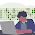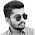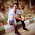# Finite State Machine that adds two integers

Q. Construct a ﬁnite-state machine or finite state automaton that adds two integers (using binary representation of integers).

Sol:
Finite Automata (FA) or Finite State Machine to add two integers can be constructed using two states:

· q0: Start state to represent carry bit is 0
·  q1: State to represent carry bit is 1

The inputs to FA will be pair of bits i.e. 00, 01, 10, and 11

The FA starts in state 1 (since carry is 0) and inputs a pair of bits. If the pair is 11, the FA outputs a 0 and switches to state 2 (since the carry is 1), where the next pair of bits is input and is added to a carry bit of 1.

Example: Consider the addition of 52 and 21
110100 - (binary representation of 52)
010101 - (binary representation of 21)

Since adding numbers is done from right to left, The first input symbol is 01, representing a 0 in the rightmost (binary) digit of 52 and a 1 in the rightmost digit of 21. The machine enters state  q(since there is no carry) and outputs a 1. The next input is 00 because both numbers have zero as the second  rightmost digit. The machine enters state q0 and outputs 0. The next input is 11. The machine enters state q1 (since the carry is 1) and outputs 0. Being in state q1 means that there is a carry from this position into the next. And the remaining bits can be worked out to get 1001001 (i.e. 73).

1.1.plz explain or more i got confused

2.3.There is a typo in the first sentence directly below the diagram. It should read as follows: The FA starts in state 0 (since carry is 0) and inputs a pair of bits. The 1 should be changed to a 0.

4.well explained!

5.grt explanation

6.What is the equivalent Moore machine?

7.awesome and ty

8.What about the last carry bit

9.last carry is write down i think